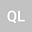loading page

The meaning of an infinitely great velocity
•## Abstract

An instantaneous velocity where clock at a moment only correponds to any arbitrary distance or position of space can not be indicated in axiom 1, but it indicates that there is only one dimensional existence,space or time, where a certain moment of clock only corresponds to a specific given length of space,not to any other distance.Further,each quantity of space and time correponds only to itself. Instead of Relavity, A velocity definition that consists of two dimensions representing relationship between space and time is not valid and there is only one dimensional space or time that is independent each other in axiom 1 .As an result,the principle of relativity and Principle of constant velocity of light are replaced by the principle of inertial system of axiom 1 and principle of universal invariant velocity of axiom 1. Unlike two dimensions whose magnutide is determined by the ratio,the magnutide of single dimension is determined by the unit values of one dimension,which indicates that an infinitely great velocity is meaningless,instead of ,there is only infinitely great space of one dimension and infinitely long time of one dimension. Further,The extensions of finite quantities of two inertial system in axiom 3 must only stay in the finite range,and do not reach infinite distance. If two such inertial systems are infinite versus finite,then it is known from axiom 3 that the change of direction means infinite great and this extension of infinite great can be defined to be inextensible.